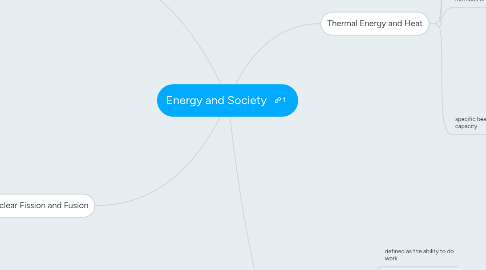# Energy and SocietyKelly Lau
Get Started. It's FreeEnergy and Society## 1. Work

### 1.2. special cases

1.2.1. positive work: force is in same direction as displacement

1.2.2. negative work: force opposes motion

1.2.3. zero work: no force and/or displacement

## 2. Energy

### 2.2. key points

2.2.1. the Law of Conservation of Energy states "Energy cannot be created or destroyed; Energy can only be converted form one form to another"

2.2.2. work is done when energy is transferred from one object to another

2.2.3. energy comes in many forms which are interchangeable

2.2.4. energy can be stored to do work later

### 2.3. energy types

2.3.1. rest mass energy

2.3.1.1. total energy of an object due to mass

2.3.2. potential energy

2.3.2.1. energy stored in an object

2.3.2.1.1. e.g. chemical, nuclear, gravitational, elastic

2.3.3. kinetic energy

2.3.3.1. energy of motion

2.3.3.1.1. e.g. sound, heat, radiant, bulk kinetic, electrical

### 2.4. common energy calculations

2.4.1. gravitational potential energy (Eg = mgΔH)

2.4.2. kinetic energy (Ek = 1/2mv^2)

2.4.3. *mass in kg*

2.4.4. remember : the total energy is the same throughout a system *top = bottom*

## 3. Thermal Energy and Heat

### 3.4. methods of heat transfer

3.4.1. conduction

3.4.1.1. the producers by which the collision of atoms and electrons transfer heat through a material or between two materials in contact (particles jiggle but do not travel)

3.4.1.1.1. e.g. copper or aluminum pots distribute heat well - no hot spots, wooden floors feel warmer than ceramic tiles, double-paned windows create air space for insulation

3.4.2. convection

3.4.2.1. transfers heat through a circulating path of fluid; circulating paths are called convection currents (particles travel)

3.4.2.1.1. e.g. cooking pasta, boiling water

3.4.3.1. transfers energy by means of electromagnetic waves and not by particles at all

3.4.3.1.1. e.g. microwaves, radio waves, X-rays, infrared, how earth gets sun's energy, emergency blankets

### 3.5. specific heat capacity

3.5.1. the amount of heat needed to raise the temperature of 1g of a substance by 1 degree celsius

3.5.2. q = mcΔt

3.5.2.1. use if phase does not change; line as slope > 0, or <0

3.5.3. *mass in g*

3.5.4. q = mΔH (vaporization)

3.5.5. q = mΔH (fusion)

3.5.6. ΔH = latent heat - the energy required to change the phase of 1g of a substance

## 4. Nuclear Fission and Fusion

### 4.2. during nuclear fusion of light elements and nuclear fission of heavy elements, a small amount of mass disappears from the nucleus

4.2.1. mass defect

4.2.1.1. turned into energy

4.2.1.1.1. E = mc^2

4.2.1.2. calculate by subtracting the mass of the products from the reactants

### 4.3. chain reaction

4.3.1. fission produces free neutrons

4.3.2. will continue unless...

4.3.2.1. minimum mass is not met; aka critical mass

4.3.2.2. free neutrons are moving too fast

4.3.2.3. neutrons are blocked; control rods used